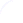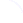Search
 Submission Procedure share: |available in:   PDF (165 kB) PS (156 kB)

get:
 Similar Docs BibTeX Write a comment
get:

DOI:   10.3217/jucs-014-06-0861

### On the Subrecursive Computability of Several Famous Constants

Dimiter Skordev (Sofia University, Bulgaria)

Abstract: For any class F of total functions in the set N of the natural numbers, we define the notion of F-computable real number. A real number α is called F-computable if there exist one-argument functions f, g and h in F such that for any n in N the distance between the rational number f(n) - g(n) over h(n) + 1 and the number α is not greater than the reciprocal of n + 1. Most concrete real numbers playing a role in analysis can be easily shown to be E3-computable (as usually, Em denotes the m-th Grzegorczyk class). Although (as it is proved in Section 5 of this paper) there exist E3-computable real numbers that are not E2-computable, we prove that π, e and other remarkable real numbers are E2-computable (the number π proves to be even L-computable, where L is the class of Skolem's lower elementary functions). However, only the rational numbers would turn out to be E2-computable according to a definition of F-computability using 2n instead of n + 1.

Keywords: Euler's constant, Grzegorczyk classes, Liouville's number, computable real number, lower elementary functions, second Grzegorczyk class, π, e

Categories: F.1.3, F.2.1, G.0, G.1.0